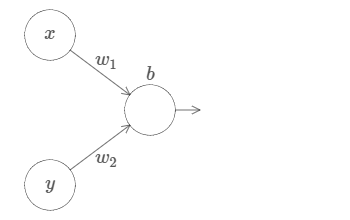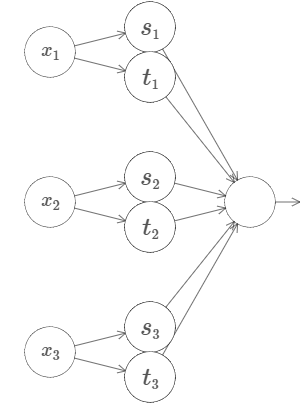# 4.3: Many input variables

$$\newcommand{\vecs}{\overset { \rightharpoonup} {\mathbf{#1}} }$$ $$\newcommand{\vecd}{\overset{-\!-\!\rightharpoonup}{\vphantom{a}\smash {#1}}}$$$$\newcommand{\id}{\mathrm{id}}$$ $$\newcommand{\Span}{\mathrm{span}}$$ $$\newcommand{\kernel}{\mathrm{null}\,}$$ $$\newcommand{\range}{\mathrm{range}\,}$$ $$\newcommand{\RealPart}{\mathrm{Re}}$$ $$\newcommand{\ImaginaryPart}{\mathrm{Im}}$$ $$\newcommand{\Argument}{\mathrm{Arg}}$$ $$\newcommand{\norm}{\| #1 \|}$$ $$\newcommand{\inner}{\langle #1, #2 \rangle}$$ $$\newcommand{\Span}{\mathrm{span}}$$ $$\newcommand{\id}{\mathrm{id}}$$ $$\newcommand{\Span}{\mathrm{span}}$$ $$\newcommand{\kernel}{\mathrm{null}\,}$$ $$\newcommand{\range}{\mathrm{range}\,}$$ $$\newcommand{\RealPart}{\mathrm{Re}}$$ $$\newcommand{\ImaginaryPart}{\mathrm{Im}}$$ $$\newcommand{\Argument}{\mathrm{Arg}}$$ $$\newcommand{\norm}{\| #1 \|}$$ $$\newcommand{\inner}{\langle #1, #2 \rangle}$$ $$\newcommand{\Span}{\mathrm{span}}$$$$\newcommand{\AA}{\unicode[.8,0]{x212B}}$$

Let's extend our results to the case of many input variables. This sounds complicated, but all the ideas we need can be understood in the case of just two inputs. So let's address the two-input case.

We'll start by considering what happens when we have two inputs to a neuron:Here, we have inputs $$x$$ and $$y$$, with corresponding weights $$w_1$$ and $$w_2$$, and a bias bb on the neuron. Let's set the weight $$w_2$$ to $$0$$, and then play around with the first weight, $$w_1$$, and the bias, $$b$$, to see how they affect the output from the neuron:As you can see, with $$w_2=0$$ the input $$y$$ makes no difference to the output from the neuron. It's as though $$x$$ is the only input.

Given this, what do you think happens when we increase the weight $$w_1$$ to $$w_1=100$$, with $$w_2$$ remaining $$0$$? If you don't immediately see the answer, ponder the question for a bit, and see if you can figure out what happens. Then try it out and see if you're right. I've shown what happens in the following movie:

----------------------------------------------------------------------------------------------------------------------------------------------------------------------------------------------------------------

Just as in our earlier discussion, as the input weight gets larger the output approaches a step function. The difference is that now the step function is in three dimensions. Also as before, we can move the location of the step point around by modifying the bias. The actual location of the step point is $$s_x≡−b/w_1$$.

Let's redo the above using the position of the step as the parameter:Here, we assume the weight on the $$x$$ input has some large value - I've used $$w_1=1000$$ - and the weight $$w_2=0$$. The number on the neuron is the step point, and the little $$x$$ above the number reminds us that the step is in the $$x$$ direction. Of course, it's also possible to get a step function in the yy direction, by making the weight on the $$y$$ input very large (say, $$w_2=1000$$, and the weight on the $$x$$ equal to $$0$$, i.e., $$w_1=0$$:The number on the neuron is again the step point, and in this case the little yy above the number reminds us that the step is in the $$y$$ direction. I could have explicitly marked the weights on the $$x$$ and $$y$$ inputs, but decided not to, since it would make the diagram rather cluttered. But do keep in mind that the little $$y$$ marker implicitly tells us that the $$y$$ weight is large, and the $$x$$ weight is $$0$$.

We can use the step functions we've just constructed to compute a three-dimensional bump function. To do this, we use two neurons, each computing a step function in the $$x$$ direction. Then we combine those step functions with weight $$h$$ and $$−h$$, respectively, where $$h$$ is the desired height of the bump. It's all illustrated in the following diagram:Try changing the value of the height, $$h$$. Observe how it relates to the weights in the network. And see how it changes the height of the bump function on the right.

Also, try changing the step point $$0.30$$ associated to the top hidden neuron. Witness how it changes the shape of the bump. What happens when you move it past the step point $$0.70$$ associated to the bottom hidden neuron?

We've figured out how to make a bump function in the $$x$$ direction. Of course, we can easily make a bump function in the $$y$$ direction, by using two step functions in the $$y$$ direction. Recall that we do this by making the weight large on the $$y$$ input, and the weight $$0$$ on the $$x$$ input. Here's the result:This looks nearly identical to the earlier network! The only thing explicitly shown as changing is that there's now little $$y$$ markers on our hidden neurons. That reminds us that they're producing $$y$$ step functions, not $$x$$ step functions, and so the weight is very large on the $$y$$ input, and zero on the $$x$$ input, not vice versa. As before, I decided not to show this explicitly, in order to avoid clutter.

Let's consider what happens when we add up two bump functions, one in the $$x$$ direction, the other in the $$y$$ direction, both of height $$h$$:To simplify the diagram I've dropped the connections with zero weight. For now, I've left in the little $$x$$ and $$y$$ markers on the hidden neurons, to remind you in what directions the bump functions are being computed. We'll drop even those markers later, since they're implied by the input variable.

Try varying the parameter $$h$$. As you can see, this causes the output weights to change, and also the heights of both the $$x$$ and $$y$$ bump functions.

What we've built looks a little like a tower function:If we could build such tower functions, then we could use them to approximate arbitrary functions, just by adding up many towers of different heights, and in different locations:Of course, we haven't yet figured out how to build a tower function. What we have constructed looks like a central tower, of height $$2h$$, with a surrounding plateau, of height $$h$$.

But we can make a tower function. Remember that earlier we saw neurons can be used to implement a type of if-then-else statement:

    if input >= threshold:
output 1
else:
output 0


That was for a neuron with just a single input. What we want is to apply a similar idea to the combined output from the hidden neurons:

    if combined output from hidden neurons >= threshold:
output 1
else:
output 0


If we choose the threshold appropriately - say, a value of $$3h/2$$, which is sandwiched between the height of the plateau and the height of the central tower - we could squash the plateau down to zero, and leave just the tower standing.

Can you see how to do this? Try experimenting with the following network to figure it out. Note that we're now plotting the output from the entire network, not just the weighted output from the hidden layer. This means we add a bias term to the weighted output from the hidden layer, and apply the sigma function. Can you find values for hh and bb which produce a tower? This is a bit tricky, so if you think about this for a while and remain stuck, here's two hints: (1) To get the output neuron to show the right kind of if-then-elsebehaviour, we need the input weights (all $$h$$ or $$−h$$) to be large; and (2) the value of bb determines the scale of the if-then-else threshold.With our initial parameters, the output looks like a flattened version of the earlier diagram, with its tower and plateau. To get the desired behaviour, we increase the parameter hh until it becomes large. That gives the if-then-else thresholding behaviour. Second, to get the threshold right, we'll choose $$b≈−3h/2$$. Try it, and see how it works!

Here's what it looks like, when we use $$h=10$$:

----------------------------------------------------------------------------------------------------------------------------------------------------------------------------------------------------------------

Even for this relatively modest value of hh, we get a pretty good tower function. And, of course, we can make it as good as we want by increasing $$h$$ still further, and keeping the bias as $$b=−3h/2$$.

Let's try gluing two such networks together, in order to compute two different tower functions. To make the respective roles of the two sub-networks clear I've put them in separate boxes, below: each box computes a tower function, using the technique described above. The graph on the right shows the weighted output from the second hidden layer, that is, it's a weighted combination of tower functions.In particular, you can see that by modifying the weights in the final layer you can change the height of the output towers.

The same idea can be used to compute as many towers as we like. We can also make them as thin as we like, and whatever height we like. As a result, we can ensure that the weighted output from the second hidden layer approximates any desired function of two variables:In particular, by making the weighted output from the second hidden layer a good approximation to $$σ^{−1}∘f$$, we ensure the output from our network will be a good approximation to any desired function, $$f$$.

What about functions of more than two variables?

Let's try three variables $$x_1,x_2,x_3$$. The following network can be used to compute a tower function in four dimensions:Here, the $$x_1,x_2,x_3$$ denote inputs to the network. The $$s_1$$,$$t_1$$ and so on are step points for neurons - that is, all the weights in the first layer are large, and the biases are set to give the step points $$s_1,t_1,s_2,…$$.The weights in the second layer alternate $$+h,−h,$$ where $$h$$ is some very large number. And the output bias is $$−5h/2$$.

This network computes a function which is $$1$$ provided three conditions are met: $$x_1$$ is between $$s_1$$ and $$t_1$$; $$x_2$$ is between $$s_2$$ and $$t_2$$; and $$x_3$$ is between $$s_3$$ and $$t_3$$. The network is $$0$$ everywhere else. That is, it's a kind of tower which is $$1$$ in a little region of input space, and $$0$$ everywhere else.

By gluing together many such networks we can get as many towers as we want, and so approximate an arbitrary function of three variables. Exactly the same idea works in $$m$$ dimensions. The only change needed is to make the output bias $$(−m+1/2)h$$, in order to get the right kind of sandwiching behavior to level the plateau.

Okay, so we now know how to use neural networks to approximate a real-valued function of many variables. What about vector-valued functions $$f(x_1,…,x_m) ∈ R^n$$ ? Of course, such a function can be regarded as just $$n$$ separate real-valued functions, $$f^1(x_1,…,x_m),f^2(x_1,…,x_m),$$ and so on. So we create a network approximating $$f^1$$, another network for$$f^2$$, and so on. And then we simply glue all the networks together. So that's also easy to cope with.

## Problem

• We've seen how to use networks with two hidden layers to approximate an arbitrary function. Can you find a proof showing that it's possible with just a single hidden layer? As a hint, try working in the case of just two input variables, and showing that: (a) it's possible to get step functions not just in the $$x$$ or $$y$$ directions, but in an arbitrary direction; (b) by adding up many of the constructions from part (a) it's possible to approximate a tower function which is circular in shape, rather than rectangular; (c) using these circular towers, it's possible to approximate an arbitrary function. To do part (c) it may help to use ideas from a bit later in this chapter.

This page titled 4.3: Many input variables is shared under a CC BY-NC 3.0 license and was authored, remixed, and/or curated by Michael Nielson via source content that was edited to the style and standards of the LibreTexts platform; a detailed edit history is available upon request.